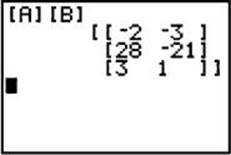﻿ ﻿MATRIX MULTIPLICATION - Matrices - Numbers and Operations - REVIEW OF MAJOR TOPICS - SAT SUBJECT TEST MATH LEVEL 2

## PART 2## REVIEW OF MAJOR TOPICS## Numbers and Operations

###3.3 Matrices### MATRIX MULTIPLICATION

Matrix multiplication takes place when two matrices, A and B, are multiplied to form a new matrix, AB. Matrix multiplication is possible only under certain conditions. Suppose A is r1 by c1 and B is r2 by c2. If c1 = r2, then AB is defined and has size r1 by c2. The entry xij of AB is the i th row of A times the j th column of B. If A and B are square matrices, BA is also defined but not generally equal to AB.

EXAMPLES

1. Evaluate AB =. A is 3 by 2, and B is 2 by 2.

The product matrix is 3 by 2, with entries xij calculated as follows:

x11 = (3)(1) + (–1)(5) = –2, the “product” of row 1 of A and column 1 of B

x12 = (3)(–2) + (–1)(–3) = –3, the “product” of row 1 of A and column 2 of B

x21 = (3)(1) + (5)(5) = 28, the “product” of row 2 of A and column 1 of B

x22 = (3)(–2) + (5)(–3) = –21, the “product” of row 2 of A and column 2 of B

x31 = (–2)(1) + (1)(5) = 3, the “product” of row 3 of A and column 1 of B

x32 = (–2)(–2) + (1)(–3) = 1, the “product” of row 3 of A and column 2 of B

Therefore, AB =. Note that BA is not defined.

Matrix calculations can be done on a graphing calculator. To define a matrix, enter 2nd MATRIX, highlight and enter EDIT, enter the number of rows followed by the number of columns, and finally enter the entries. The figure below shows the result of these steps for matrices A and B of Example 1.To find the product, enter 2nd MATRIX/NAMES/[A], which returns [A] to the home screen. Also enter 2nd MATRIX/NAMES/[B], which returns [B] to the home screen. Hit ENTER again to get the product.Square matrices of the same size can always be multiplied. However, matrix multiplication is not commutative.

2. If, evaluate AB and BA.EXERCISES

Use A = [-2 -1 5 9] and B =for questions 1 and 2.

1. The product AB =

(A)(B) [–37 21]

(C)(D)(E) product is not defined

2. The first row, second column of the productis

(A) –5x – 3

(B) –x – 3

(C) 1 – x2

(D) 4x

(E) 2x + 2

3. If, and AX = B , then the size of X is

(A) 3 rows, 3 columns

(B) 3 rows, 2 columns

(C) 2 rows, 2 columns

(D) 2 rows, 3 columns

(E) cannot be determined

4. The chart below shows the number of small and large packages of a certain brand of cereal that were bought over a three-day period. The price of a small box of this brand is \$2.99, and the price of a large box is \$3.99. Which of the following matrix expressions represents the income, in dollars, received from the sale of cereal each of the three days?(A)(B)(C)(D)(E)﻿